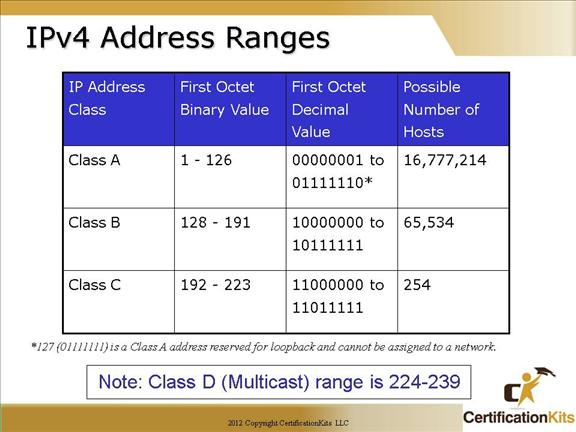The number of possible hosts is a Class A address are much greater than the number of possible hosts in a Class C address.  Fortunately with the use of Variable Length Subnet Masks (VLSM), classfull boundaries can be removed to make better use of address space.  In order to properly route IP packets utilizing VLSM, a classless routing protocol like OSPF, EIGRP or RIP version 2 must be used.  Routing protocols such as RIP version 1 or IGRP do not recognize VLSM as they are classful routing protocols.

The designers of the IP address scheme said that the first bit of the first byte in a Class A network address must always be off, or 0. This means a Class A address must be between 0 and 126 (127 is reserved for loopback address).

In a Class B network, the RFCs state that the first bit of the first byte must always be turned on, but the second bit must always be turned off. If you turn the other six bits all off and then all on, you will find the range for a Class B network:

10000000 = 128

10111111 = 191

For Class C networks, the RFCs define the first two bits of the first octet always turned on, but the third bit can never be on. Following the same process as the previous classes, convert from binary to decimal to find the range. Here’s the range for a Class C network:

11000000 = 192

11011111 = 223

So, if you see an IP address that starts at 192 and goes to 223, you’ll know it is a Class C IP address.

Cisco CCNA Binary to Decimal Conversion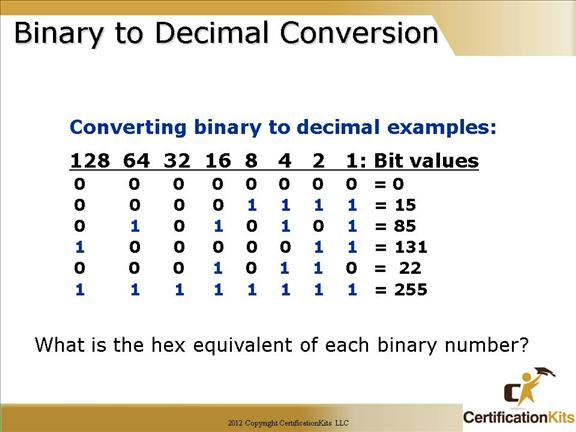We discussed this in chapter 1, but it is important enough to review at this point:

It’s pretty simple really. The digits used are limited to either a 1(one) or a 0 (zero), with each digit being called one bit.  Typically, you count either four or eight bits together, with these being referred to as a nibble or a byte, respectively.

What interests us in binary numbering is the value represented in a decimal format—the typical decimal format being our base ten number scheme we’ve all used since kindergarten.

The binary numbers are placed in a value spot; starting at the right and moving left, with each spot having double the value of the previous spot.

128  64  32  16  8  4  2  1

So explaining a couple of examples on the slide are as follows:

85 equals 01010101 which equates to 64 + 16 + 4 + 1 = 85

131 equals 10000011 which equates to 128 + 2 + 1 = 131 simple really.

Cisco CCNA Binary (Cont.)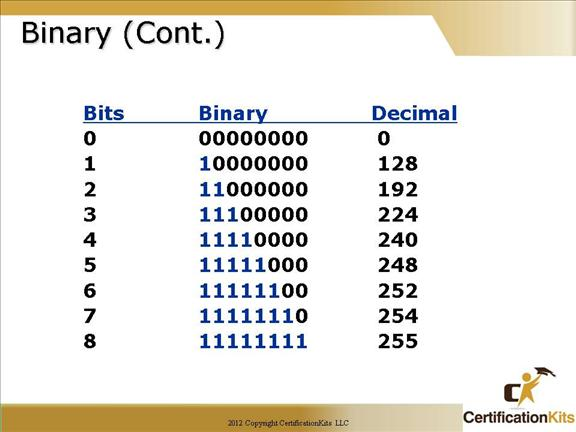Here is a binary chart that is best just to memorize.  You will need to know this off the top of your head as we delve deeper into subnetting and when you take the CCNA test.  Notice that it is no more than taking the information just learned on the previous slide and performing a little addition.  Remember the bit values were as follows:

128  64  32  16  8  4  2  1

So 11000000 equates to 128 + 64 = 192

11100000 equates to 128 + 64 + 32 = 224

11110000 equates to 128 + 64 + 32 + 16 = 240

11111000 equates to 128 + 64 + 32 + 16 + 8 = 248

11111100 equates to 128 + 64 + 32 + 16 + 8 + 4 = 252

11111110 equates to 128 + 64 + 32 + 16 + 8 + 4 + 2 = 254

11111111 equates to 128 + 64 + 32 + 16 + 8 + 4 + 2 + 1 = 255

Cisco CCNA Math to memorize!Block sizes will be discussed in more detail later.

Cisco CCNA Powers of 2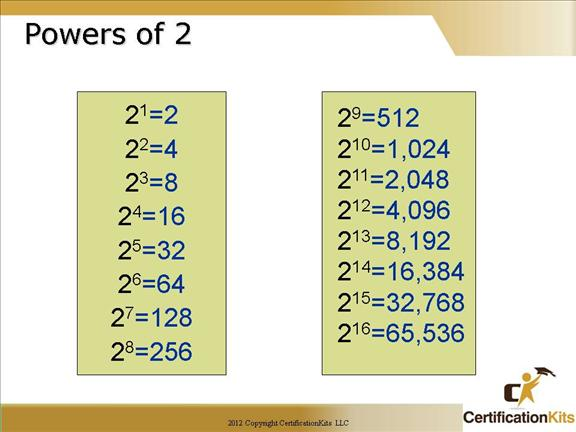Powers of 2 are important to understand and memorize for use with IP subnetting.

To review powers of 2, remember that when you see a number with another number to its upper right (called an exponent), this means you should multiply the number by itself as many times as the upper number specifies.

For example, 23 is 2 ´ 2 ´ 2, which equals 8.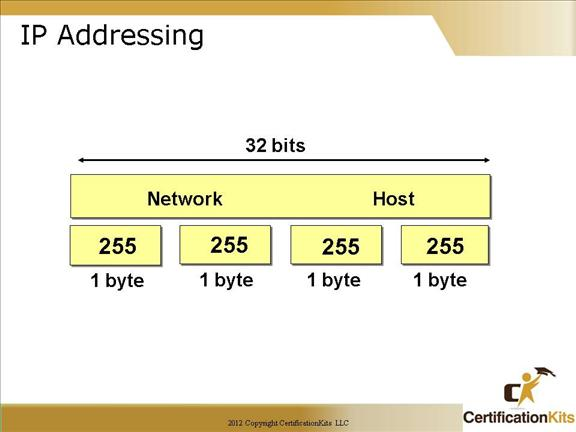An IP address consists of 32 bits of information.  These bits are divided into four sections, referred to as octets or bytes, each containing 1 byte (8 bits).   The address is logically separated into a network portion and a host portion.  The subnet mask defines where the network portion ends and the host portion begins.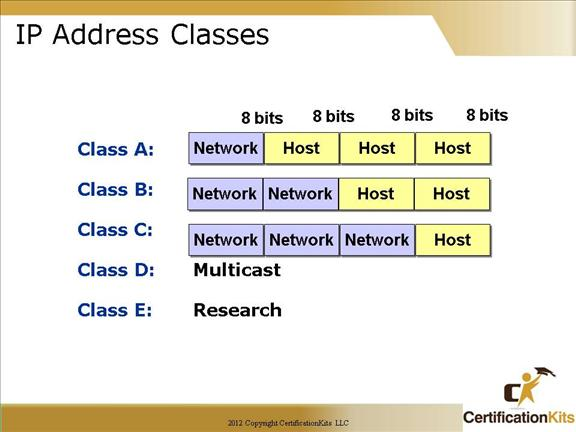The designers of the Internet decided to create classes of networks based on network size. For the small number of networks possessing a very large number of nodes, they created the rank Class A network.

At the other extreme is the Class C network, which is reserved for the numerous networks with a small number of nodes.

The class distinction for networks between very large and very small is predictably called the Class B network.

Subdividing an IP address into a network and node address is determined by the class designation of one’s network.

With the advent of Variable Length Subnet Masks (VLSM), the distinction between the different classes of IP addresses are not as important as they used to be.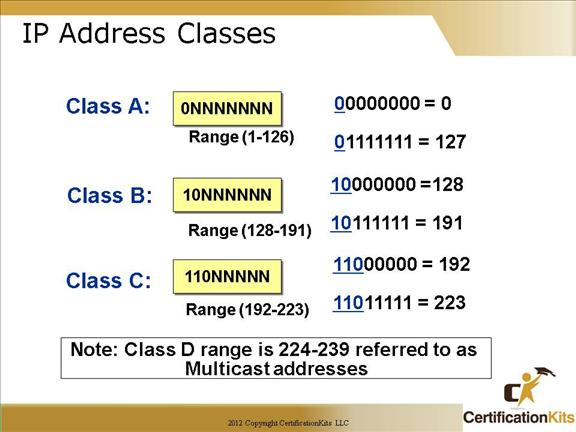The designers of the IP address scheme said that the first bit of the first byte in a Class A network address must always be off, or 0. This means a Class A address must be between 0 and 127.

In a Class B network, the RFCs state that the first bit of the first byte must always be turned on, but the second bit must always be turned off. If you turn the other six bits all off and then all on, you will find the range for a Class B network:

10000000 = 128

10111111 = 191

For Class C networks, the RFCs define the first two bits of the first octet always turned on, but the third bit can never be on. Following the same process as the previous classes, convert from binary to decimal to find the range. Here’s the range for a Class C network:

11000000 = 192

11011111 = 223

So, if you see an IP address that starts at 192 and goes to 223, you’ll know it is a Class C IP address.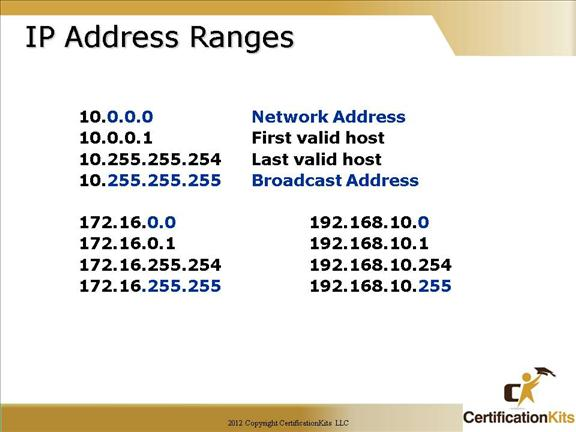Here’s an example of how to figure out the valid host IDs in a Class A network address:

All host bits off is the network address: 10.0.0.0.

The valid hosts are the number in between the network address and the broadcast address: 10.0.0.1 through 10.255.255.254.

Notice that 0s and 255s can be valid host IDs. All you need to remember when trying to find valid host addresses is that the host bits can’t all be turned off (defines network address) or all be on (defines broadcast address) at the same time.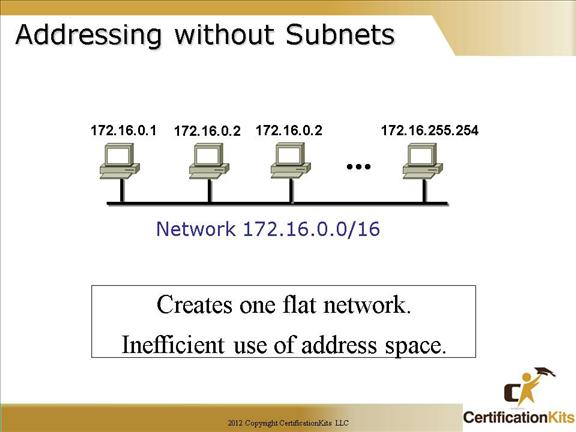Without creating subnetworks, all hosts would be on one large network. Not good…really not good.  This type of network creates one large broadcast domain.  It is not scalable.  Routers are used to break up broadcast domains and allow for communication between different ip subnets.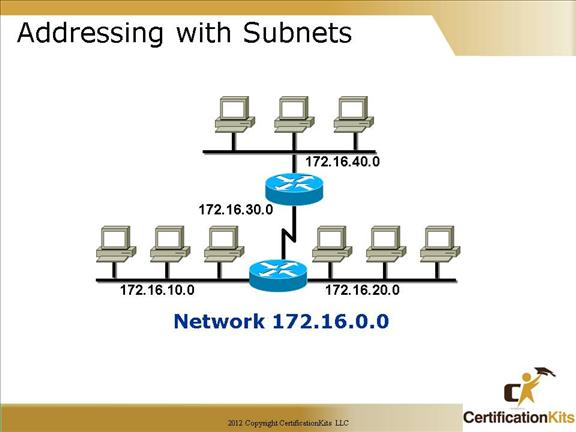There are lots of reasons to utilize ip subnetting. Some of the benefits include:

Reduced network traffic

Optimized network performance

Simplified management

Facilitates spanning of large geographical distances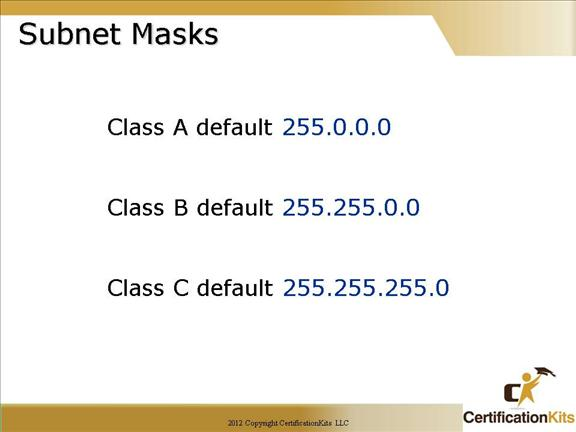For the subnet address scheme to work, every machine on the network must know which part of the host address will be used as the subnet address.

This is accomplished by assigning a subnet mask to each machine. A subnet mask is a 32-bit value that allows the recipient of IP packets to distinguish the network ID portion of the IP address from the host ID portion of the IP address.

The network administrator creates a 32-bit subnet mask composed of 1s and 0s. The 1s in the subnet mask represent the positions that refer to the network or subnet addresses.  Not all networks need subnets, meaning they use the default subnet mask. This is basically the same as saying that a network doesn’t have a subnet address.  0s in the mask indicate that bit position corresponds to the host portion of the ip address.

For example a subnet mask of 255.255.255.240 (or a /28) indicates that the first 28 bits of the ip address correspond to the network/subnet portion while the last four bits correspond to the host portion.